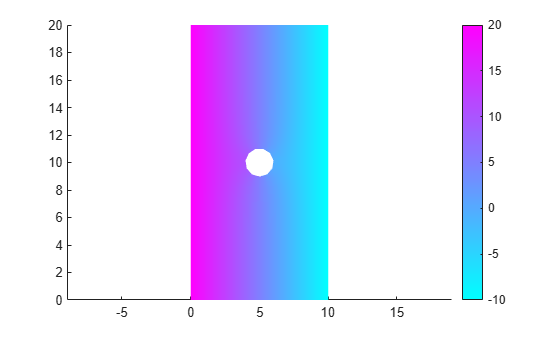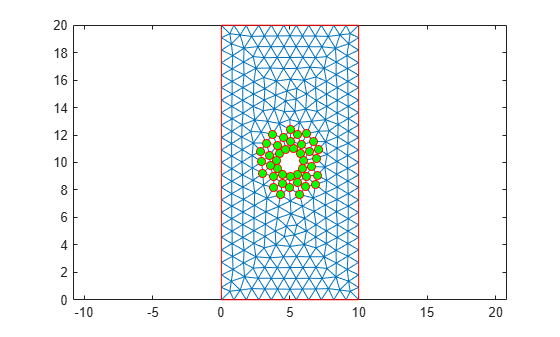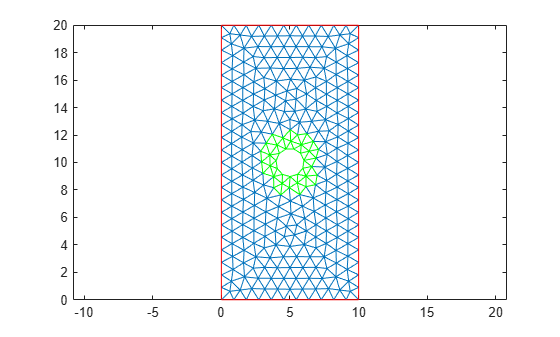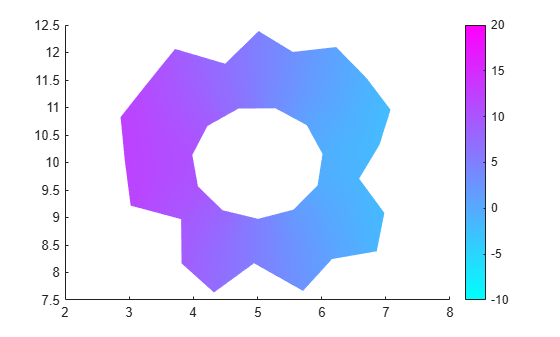# Find Mesh Elements and Nodes by Location

Partial Differential Equation Toolbox™ allows you to find mesh elements and nodes by their geometric location or proximity to a particular point or node. This example works with a group of elements and nodes located within the specified bounding disk.

`thermalmodel = createpde('thermal','steadystate');`

Import and plot the geometry.

```importGeometry(thermalmodel,'PlateHolePlanar.stl'); pdegplot(thermalmodel,'FaceLabels','on', ... 'EdgeLabels','on')```Assign the thermal conductivity of the material.

`thermalProperties(thermalmodel,'ThermalConductivity',1);`

Apply a constant temperature of ${20}^{\circ }\mathit{C}$ to the left edge and a constant temperature of ${-10}^{\circ }\mathit{C}$to the right edge. All other edges are insulated by default.

```thermalBC(thermalmodel,'Edge',4,'Temperature',20); thermalBC(thermalmodel,'Edge',1,'Temperature',-10);```

Generate a mesh and solve the problem. For this example, use a linear mesh to better see the nodes on the mesh plots. Additional nodes on a quadratic mesh make it difficult to see the plots in this example clearly.

```mesh = generateMesh(thermalmodel, ... 'GeometricOrder','linear'); thermalresults = solve(thermalmodel);```

The solver finds the temperatures and temperature gradients at all nodal locations. Plot the temperatures.

```pdeplot(thermalmodel,'XYData',thermalresults.Temperature) axis equal```Suppose you need to analyze the results around the center hole more closely. First, find the nodes and elements located next to the hole by using the `findNodes` and `findElements` functions. For example, find nodes and elements located within the radius of 2.5 from the center [5 10].

```Nr = findNodes(mesh,'radius',[5 10],2.5); Er = findElements(mesh,'radius',[5 10],2.5);```

Highlight the nodes within this radius on the mesh plot using a green marker.

```figure pdemesh(thermalmodel) hold on plot(mesh.Nodes(1,Nr),mesh.Nodes(2,Nr), ... 'or','MarkerFaceColor','g')```Find the minimal and maximal temperatures within the specified radius.

```[Temps_disk] = thermalresults.Temperature(Nr); [T_min,index_min] = min(Temps_disk); [T_max,index_max] = max(Temps_disk); T_min```
```T_min = -2.1698 ```
`T_max`
```T_max = 12.2420 ```

Find the IDs of the nodes corresponding to the minimal and maximal temperatures. Plot these nodes on the mesh plot.

```nodeIDmin = Nr(index_min); nodeIDmax = Nr(index_max); figure pdemesh(thermalmodel) hold on plot(mesh.Nodes(1,nodeIDmin), ... mesh.Nodes(2,nodeIDmin), ... 'or','MarkerFaceColor','b') plot(mesh.Nodes(1,nodeIDmax), ... mesh.Nodes(2,nodeIDmax), ... 'or','MarkerFaceColor','r')```Now highlight the elements within the specified radius on the mesh plot using a green marker.

```figure pdemesh(thermalmodel) hold on pdemesh(mesh.Nodes,mesh.Elements(:,Er), ... 'EdgeColor','green')```Show the solution for only these elements.

```figure pdeplot(mesh.Nodes,mesh.Elements(:,Er), ... 'XYData',thermalresults.Temperature)```## SupportGet trial now